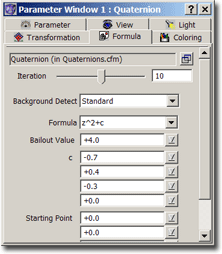### Quaternion – Formula ParametersThe formula tab lets you specify the iteration formula to use for the fractal. Assign such a formula by double clicking onto the formula in the compiler window or by selecting it and pressing the “Set”-button.

You then can change the parameters of this formula.

For more information about setting parameters of your formula see Setting Formula Parameters.

• Iteration

Here you can set the beloved “number of iteration”-parameter. It specifies how many iterations ChaosPro should perform. The higher the value, the more exact the quaternion, the slower the calculation.

Quaternions look best with low iteration values. The smaller the iteration values, the smoother the quaternion! Most of the time very low values will lead to a distorted ball. The higher the iteration value, the more details appear, but the object will get holes.

• Background Detect

A new parameter introduced in ChaosPro 3.1 is the “Background Detection”: It speeds up the calculation by guessing where the background is. The background areas are the most computational intensiv areas, thus applying some logic to detect the background has a great impact on the calculation time.